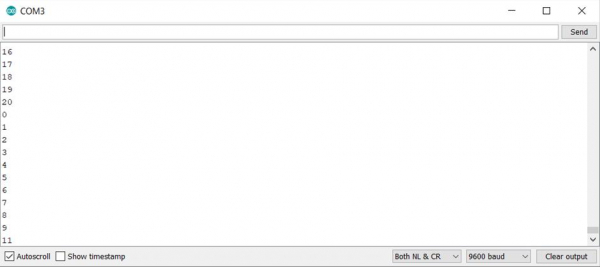# For and While loops in Arduino

The for and while loops in Arduino follow the C language syntax.

The syntax for the for loop is −

## Syntax

for(iterator initialization; stop condition; increment instruction){
//Do something
}

## Example

for(int i = 0; i< 50; i++){
//Do something
}

Similarly, the syntax for the while loop is −

## Syntax

while(condition){
//Do something
}

## Example

int i = 0
while(i < 50){
//Do something
i = i+1;
}

The following example will illustrate the working of for and while loops in an Arduino program.

## Example

void setup() {
Serial.begin(9600);
}
void loop() {
// put your main code here, to run repeatedly:
int i = 0;
for(i = 0; i< 10; i++){
Serial.println(i);
}

while(i < 20){
i = i+1;
Serial.println(i);
}
}

Note that we defined the integer i outside the for loop. Had I written for(int i = 0; i< 10; i++), then the scope of the variable i would have been restricted to the for loop only, and the while loop would not have been able to access it.

The Serial Monitor output of the above program is shown below −

## OutputAs you can see, the two loops are sharing the variable, i.

In order to write infinite loops, you can use the following syntax for for loops −

## Syntax

for(;;){
//Do something continuously
}

And the following for the while loops −

## Syntax

while(1){
//Do something continuously
}